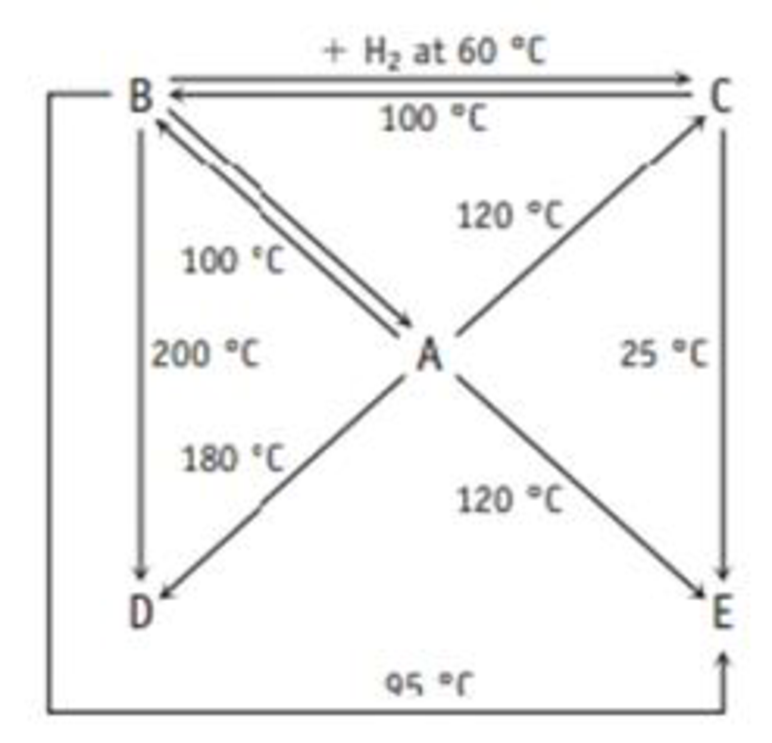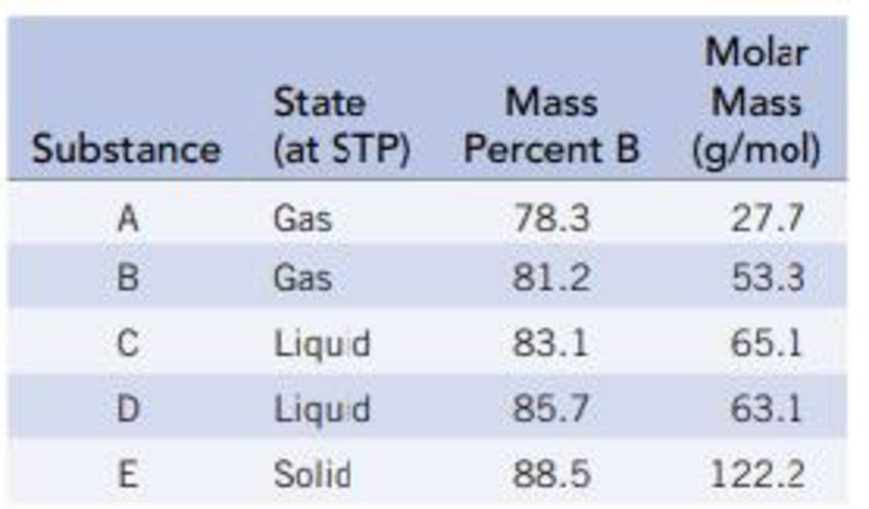Chapter 21, Problem 107GQ

Chapter
Section
Textbook Problem

A Boron and hydrogen form an extensive family of compounds, and the diagram below shows how they are related by reaction.The following table gives the weight percent of boron in each of the compounds. Derive the empirical and molecular formulas of compounds A-E.Interpretation Introduction

Interpretation: To determine the empirical and molecular formula of given compounds A-E.

Concept introduction:

The empirical formula of a chemical compound is the simplest positive integer ratio of atoms present in a compound.

A molecular formula shows the total number of atoms in a molecule but not their structural arrangement.

Explanation

Boron and hydrogen form an extensive family of compounds. Substance A-E contains boron and hydrogen atoms.

The empirical and molecular formula of given compounds A-E is calculated below.

Given:

Substance A is a gaseous compound contains 78.3% by mass of boron and 21.7% by mass of hydrogen. The molecular weight of boron is 10.8gmol1 and the molecular weight of hydrogen atom is 1.008gmol1.

The empirical formula of substance A is calculated as,

Convert the mass of boron and hydrogen into moles using molar mass of boron and hydrogen respectively.

78.3g10.8gmol1=7.25molboron21.7g1.008gmol1=21.5molhydrogen

Divide each mole value by the smallest number of moles calculated. Round off to the nearest whole number.

7.25molboron7.25mol=1.0021.5molhydrogen7.25mol3.00

Thus, the empirical formula of compound A is BH3.

The empirical formula molar mass of compound A is 13.82gmol1 and the molecular formula molar mass of compound A is 27.7gmol1.

Divide the molecular formula mass by the empirical formula mass,

27.7gmol113.8gmol1=2.00

Multiply each of the subscripts within the empirical formula of substance A by the number calculated above.

Thus, the molecular formula of substance A is 2(BH3) that is B2H6.

Substance B is a gaseous compound contains 81.2% by mass of boron and 18.8% by mass of hydrogen. The molecular weight of boron is 10.8gmol1 and the molecular weight of hydrogen atom is 1.008gmol1.

The empirical formula of substance B is calculated as,

Convert the mass of boron and hydrogen into moles using molar mass of boron and hydrogen respectively.

81.2g10.8gmol1=7.52molboron18.8g1.008gmol1=18.65molhydrogen

Divide each mole value by the smallest number of moles calculated. Round off to the nearest whole number.

7.52molboron7.52mol=1.0018.65molhydrogen7.52mol=2.5

Thus, the empirical formula of compound B is BH2.5.

The empirical formula molar mass of compound B is 12.82gmol1 and the molecular formula molar mass of compound B is 53.3gmol1.

Divide the molecular formula mass by the empirical formula mass,

53.3gmol112.8gmol14.00

Multiply each of the subscripts within the empirical formula of substance B by the number calculated above.

Thus, the molecular formula of substance B is 4(BH2.5) that is B4H10.

Substance C is a liquid compound contains 83.1% by mass of boron and 16.9% by mass of hydrogen. The molecular weight of boron is 10.8gmol1 and the molecular weight of hydrogen atom is 1.008gmol1.

The empirical formula of substance C is calculated as,

Convert the mass of boron and hydrogen into moles using molar mass of boron and hydrogen respectively.

83.1g10.8gmol1=7.69molboron16.9g1.008gmol1=16.76molhydrogen

Divide each mole value by the smallest number of moles calculated. Round off to the nearest whole number.

7.69molboron7

Still sussing out bartleby?

Check out a sample textbook solution.

See a sample solution

The Solution to Your Study Problems

Bartleby provides explanations to thousands of textbook problems written by our experts, many with advanced degrees!

Get Started

Find more solutions based on key concepts